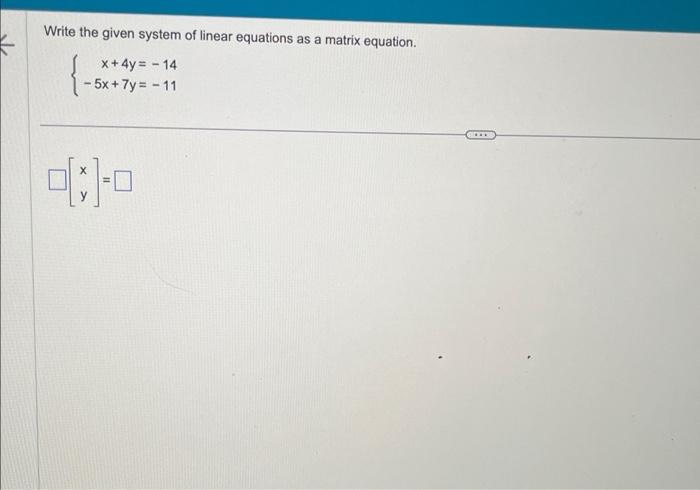Home / Expert Answers / Other Math / 15-write-the-given-system-of-linear-equations-as-a-matrix-equation-left-begin-array-r-x-4-y-pa183

# (Solved): 15 Write the given system of linear equations as a matrix equation. $\left\{\begin{array}{r} x+4 y ... 15Write the given system of linear equations as a matrix equation. \[ \left\{\begin{array}{r} x+4 y=-14 \\ -5 x+7 y=-11 \end{array}\right.$ $\left[\begin{array}{l} x \\ y \end{array}\right]=$

We have an Answer from Expert